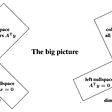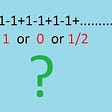# How to Calculate the Volume of a Cube

To calculate the volume of a cube with a side length `a`, use the following formula:

In other words, multiply the side length of the cube by itself three times.

But what if the sides are not equally long? Then we are talking about a cuboid, not a cube.

# How to Calculate Volume of a Cuboid

To calculate the volume of a cuboid, multiply the side lengths by one another using this formula:

Where `a`,`b`, and `c` are lengths of the sides of the cube.

# Examples

## Example 1

Find the volume of a cube with the side length `2`.

Solution. Let’s substitute the side length into the cube volume equation:

Answer. The volume of this cube is 8.

## Example 2

Find the volume of a cuboid with side lengths `1`, `2`, `3`.

Solution. Let’s substitute the side lengths into the cuboid volume equation:

Answer. The volume of this cuboid is `6`.

## Example 3

What is the side length of a cube with a volume of `10`?

Solution. To figure out the side length, you need to solve the variable `a` in the cube volume equation.

To do this, take the 3rd root of both sides of the equation. This gives you:

Answer. The side length of a cube of volume 10 is approximately 2.15.

## Example 4

On a rainy day, a soccer field of size `110m x 80m` gets `1cm` of percipitation. How many liters of water is there on the field?

Solution. The water layer and the field form a cuboid. The height of the cuboid is 1cm. This needs to be converted to meters to get the right answer. `1cm = 0.01m`. Now you can plug in the cuboids measurements into the cuboid volume equation.

Answer. The soccer pitch collected `88 000` liters of water on a rainy day.

# Conclusion

Thanks for reading, I hope you enjoy it!

--

--

--

## More from Artturi Jalli

I’m a tech expert, entrepreneur, and software engineer from Finland. I write about programming, tech, and science. Visit my blog at www.codingem.com.

Love podcasts or audiobooks? Learn on the go with our new app.

## Powers and the exponential function## 4D Result Live — How to win in Magnum 4d## Linear Algebra — Enlightening Layman’s view## 4d Result Live — How to win 4d## How To Refute Grandi’s Series## Artturi Jalli

I’m a tech expert, entrepreneur, and software engineer from Finland. I write about programming, tech, and science. Visit my blog at www.codingem.com.

## Brute-force code for Isomorphisms## How Far Is the Horizon